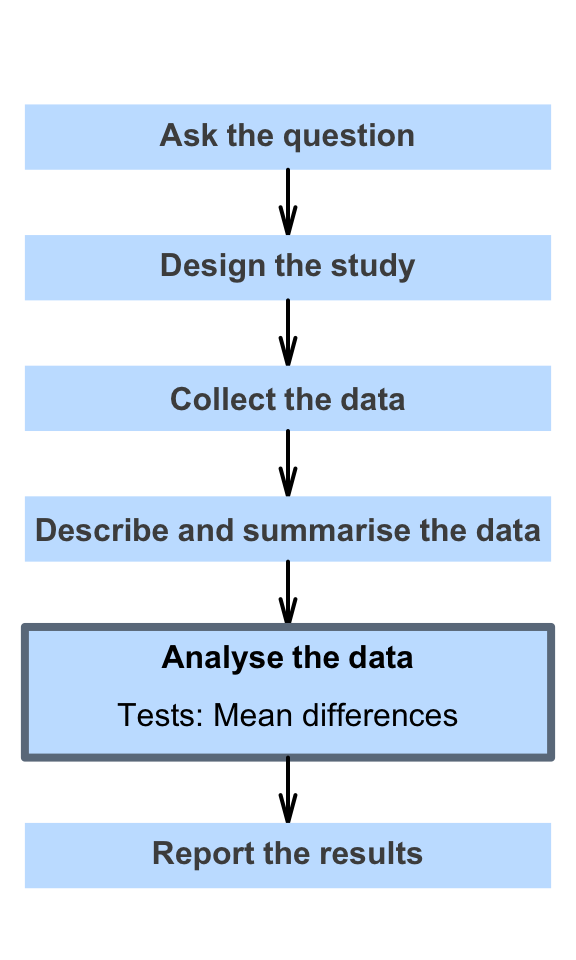# 29 Hypothesis tests for the mean difference (paired data)

So far, you have learnt to ask a RQ, identify different ways of obtaining data, design the study, collect the data describe the data, summarise data graphically and numerically, understand the tools of inference, and to form confidence intervals.

In this chapter, you will learn about hypothesis tests for the mean difference (i.e., for paired data). You will learn to:

• conduct hypothesis tests for the mean difference with paired data
• determine whether the conditions for using these methods apply in a given situation.